Algebra 2 6-2 Complete Lesson: Multiplying and Dividing Radical Expressions
starstarstarstarstarstarstarstarstarstar
4 (1 rating)
by Matthew Richardson
| 27 Questions
Note from the author:
A complete formative lesson with embedded slideshow, mini lecture screencasts, checks for understanding, practice items, mixed review, and reflection. I create these assignments to supplement each lesson of Pearson's Common Core Edition Algebra 1, Algebra 2, and Geometry courses. See also mathquest.net and twitter.com/mathquestEDU.The outlined content above was added from outside of Formative.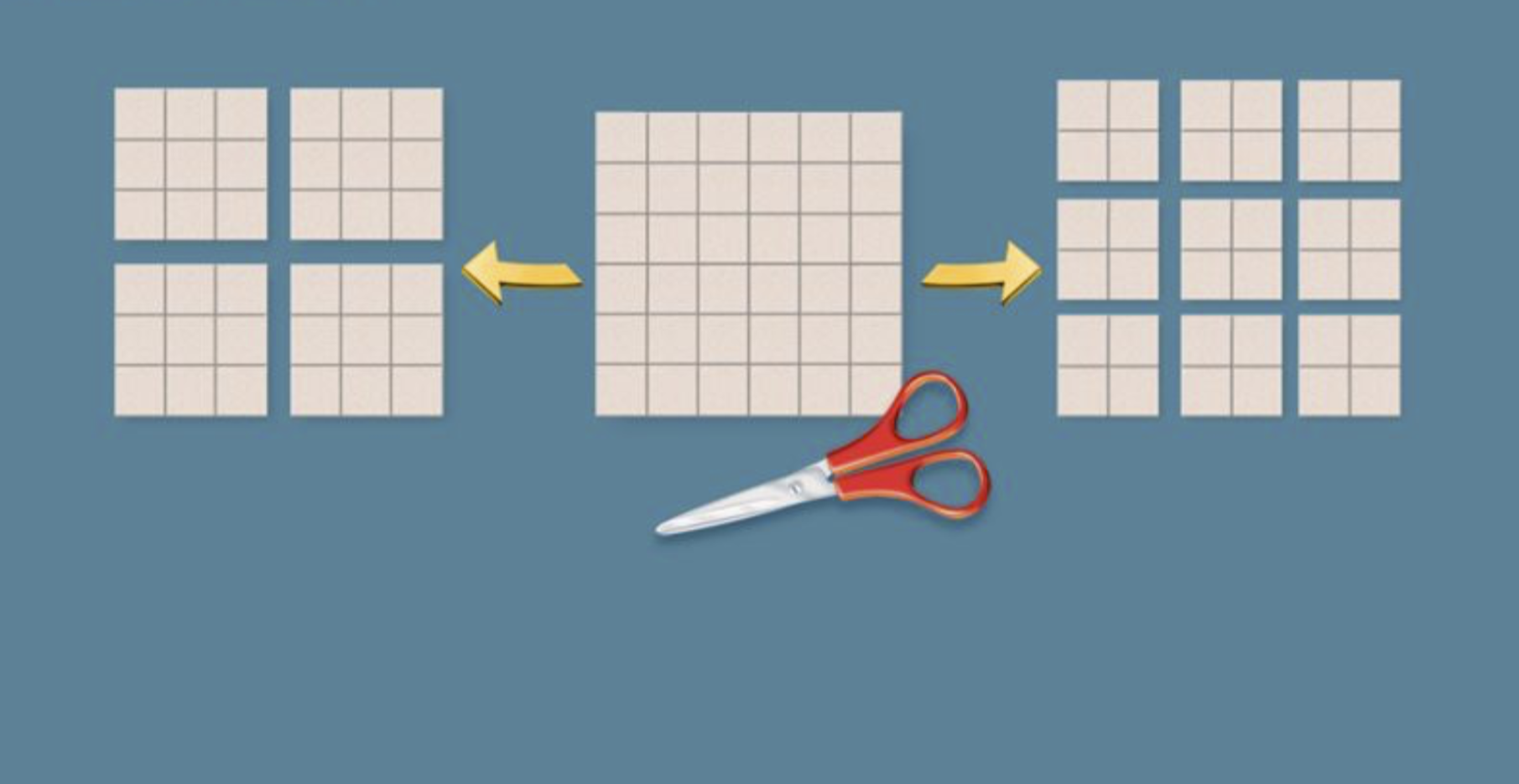1
2
1
10 pts
Solve It! You can cut the 36-square into four 9-squares or nine 4-squares. Which other n-square can you cut into sets of smaller squares in two ways?
An 18-square
A 49-square
A 64-square
2
10 pts
Solve It! Is there a square you can cut into smaller squares in three ways? Explain.
Yes, any n-square where n is the product of three perfect squares can be cut into smaller squares in three ways.
No, this only works when n is a perfect square and only creates two ways in which to divied the n-square into squares.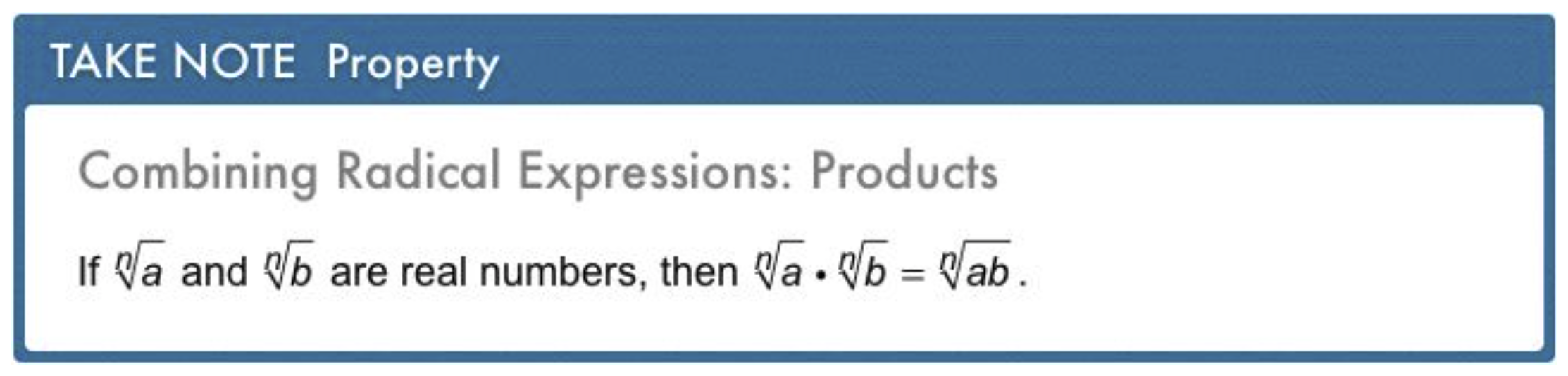3
10 pts
Problem 1 Got It? Can you simplify the expression? If so, simplify. If not, explain why not.

Yes;

No; The indexes are different.
Yes;

4
10 pts
Problem 1 Got It? Can you simplify the expression? If so, simplify. If not, explain why not.

No; The indexes are different.
Yes;

Yes;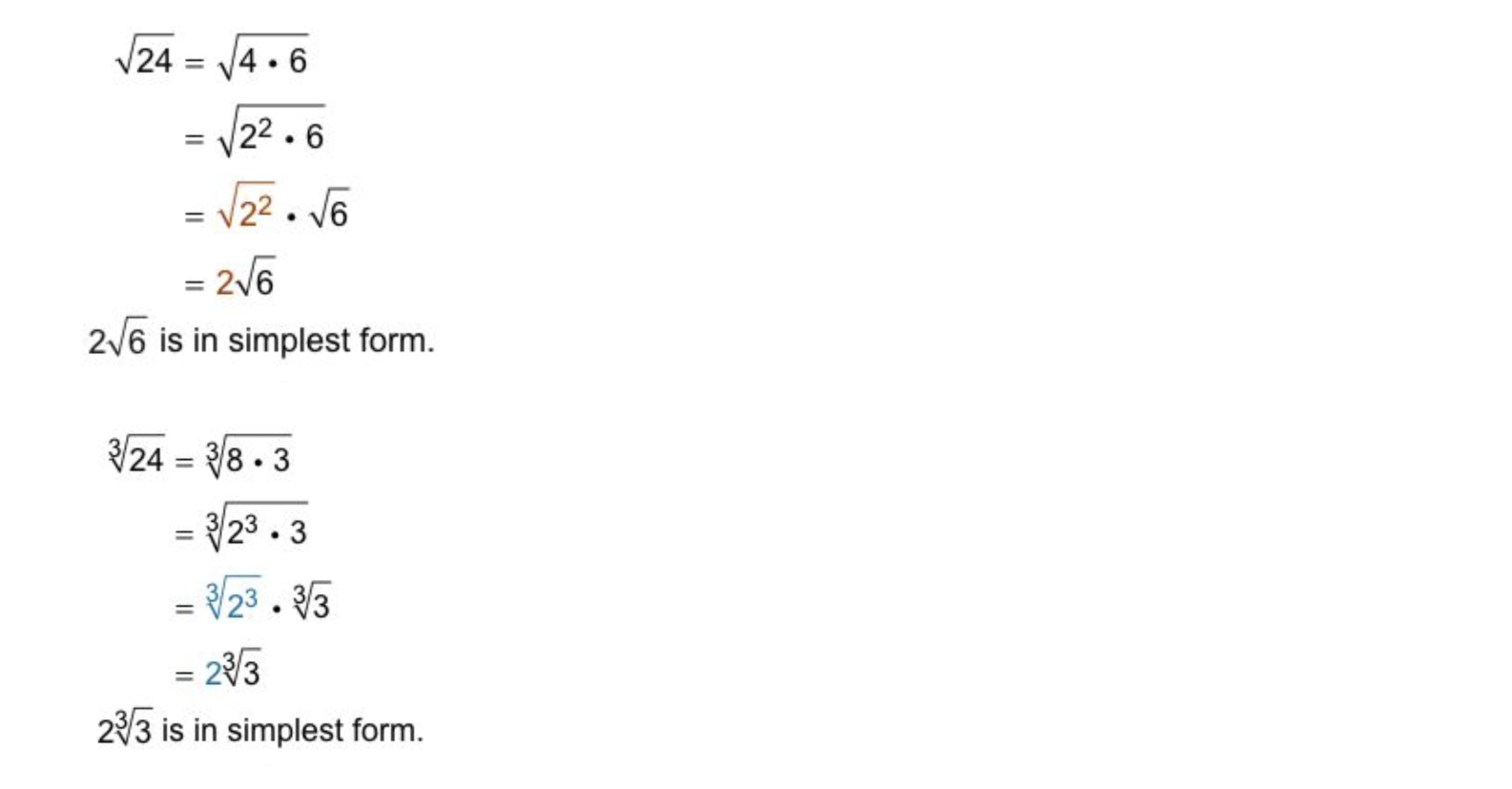5
5
10 pts
Problem 2 Got It?
A
B
C
D6
6
10 pts
Problem 3 Got It?
A
B
C
D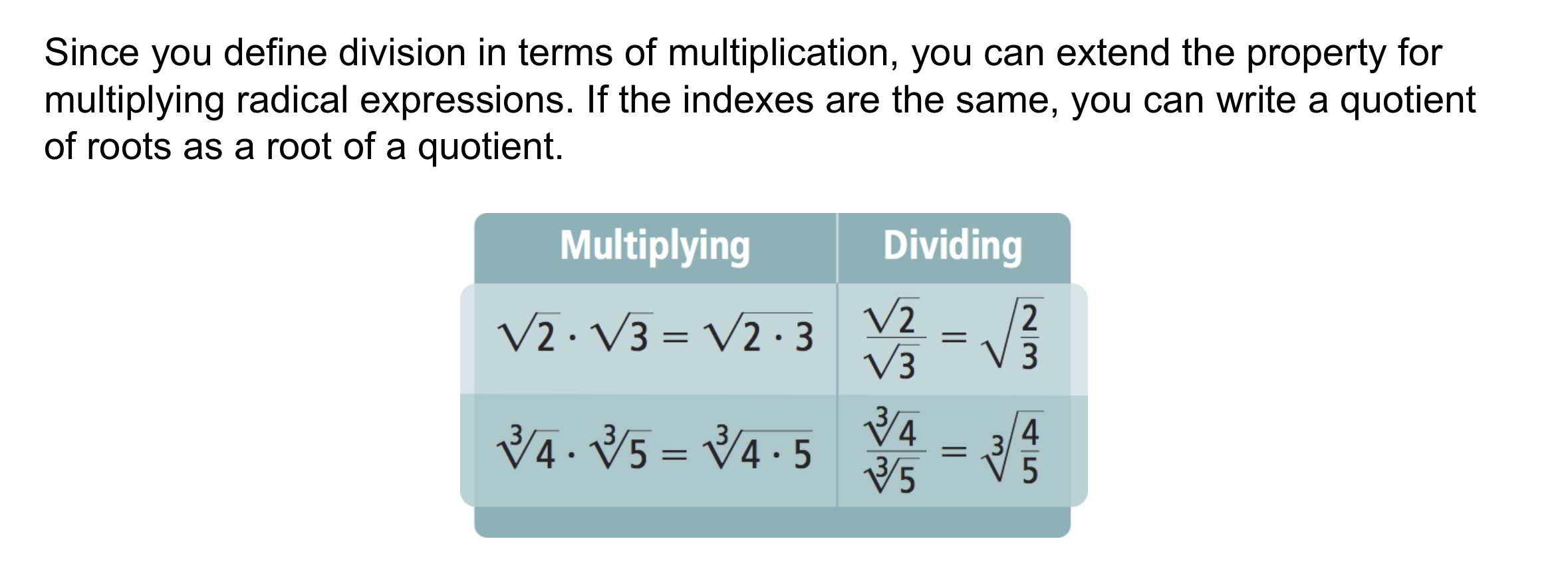7
10 pts
Problem 4 Got It? What is the simplest form of the expression?8
10 pts
Problem 5 Got It? What is the simplest form of the expression?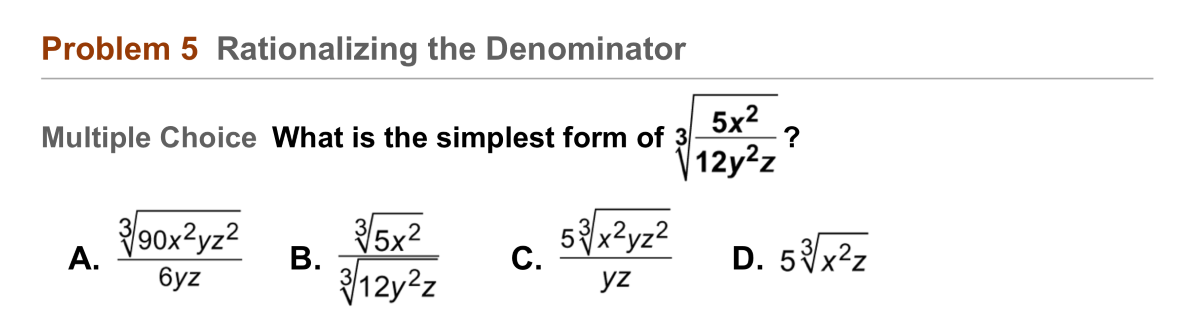9
9
10 pts
Problem 5 Got It? Which answer choices in Problem 5 could have been eliminated immediately? Explain. Select all that apply.
A; There is a cube root in the numerator.
D; There is no y in the expression.
C; There is a cube root in the denominator.
B; There is a cube root in the denominator.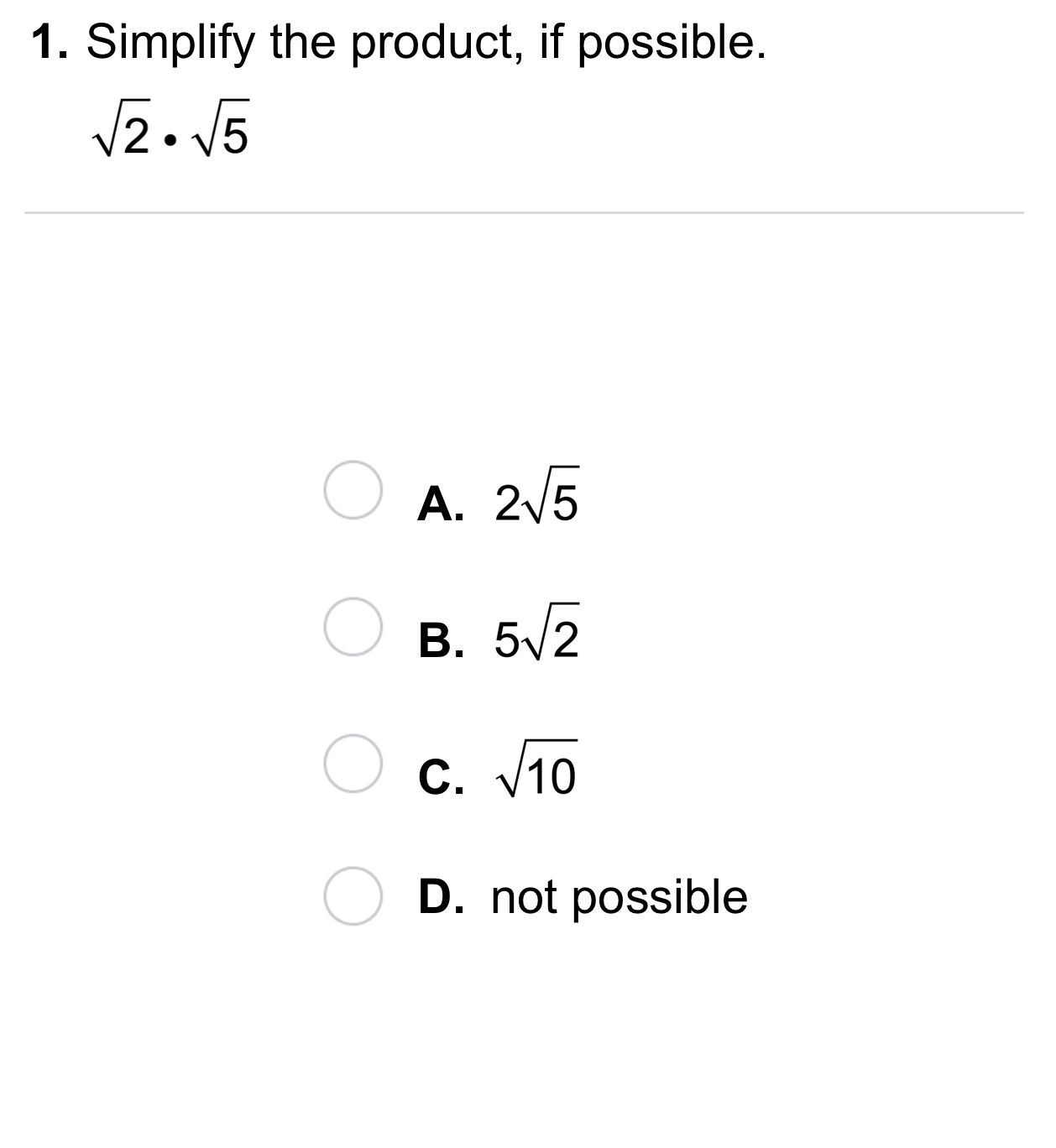10
10
10 pts
A
B
C
D11
11
10 pts
A
B
C
D12
12
10 pts
A
B
C
D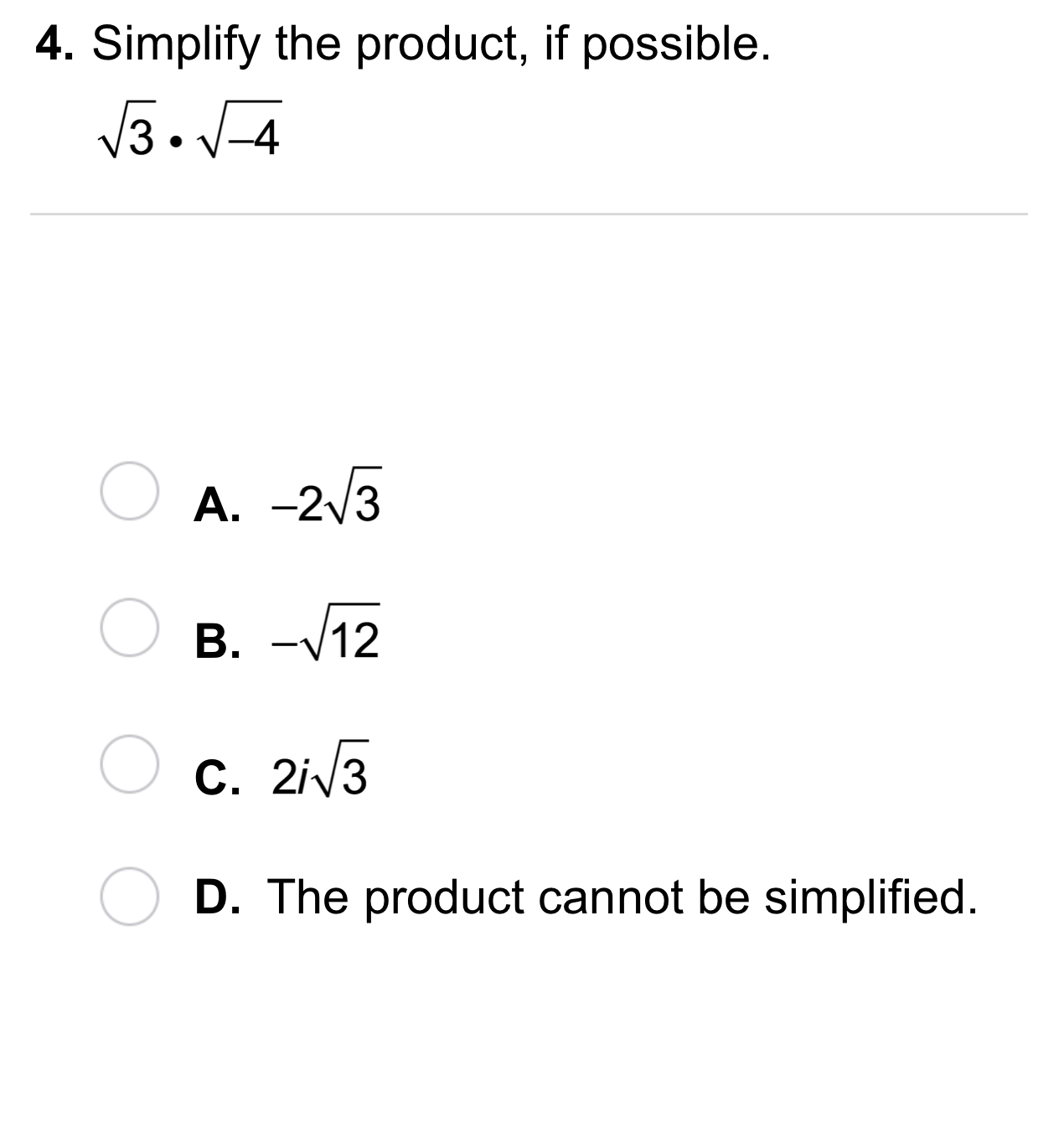13
13
10 pts
A
B
C
D14
14
10 pts
A
B
C
D15
15
10 pts
A
B
C
D16
10 pts
Vocabulary: Write the simplest form of the expression.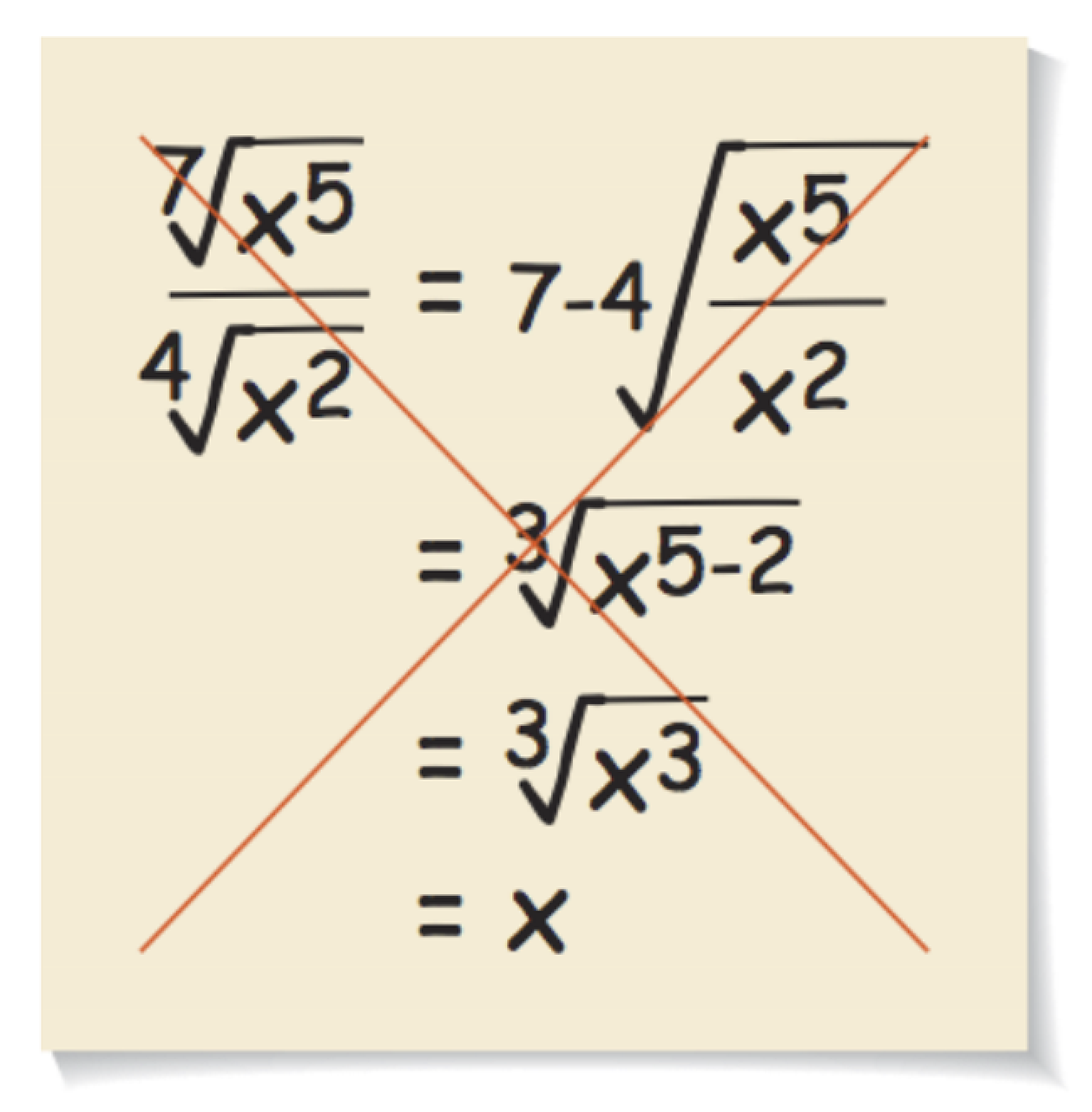18
18
10 pts
Error Analysis: Explain the error in this simiplification of radical expressions.19
10 pts
Review Lesson 6-1: Simplify the radical expression. Use absolute value symbols when needed.

20
30 pts
Review Lesson 5-4: Divide using synthetic division. Show each step.

21
10 pts
Review Lesson 4-6: Complete each square.
• 16
• 25
• 4
• + 10x + __?__
• - 10x + __?__
• + 4x + __?__
• + 8x + __?__
22
10 pts
Review Lesson 4-8: Write the quotient as a complex number in the form a ± bi.23
10 pts
Vocabulary Review: Categorize each statement as true or false.
• All mathematical expressions can be written as an equivalent expression with a denominator of 1.
• An real number can have a denominator equal to 0.
• The expression above a fraction bar is called the denominator.
• Multiplying both the numerator and denominator by the same nonzero number results in an equivalent fraction.
• True
• False
24
10 pts
Vocabulary Review: Consider the three rational expressions below.
Drag and drop the expressions on the left to identify them as a numerator or denominator.
• 5
• 6
• -5
• 16
• +
• c - 16
• Numerators
• Denominators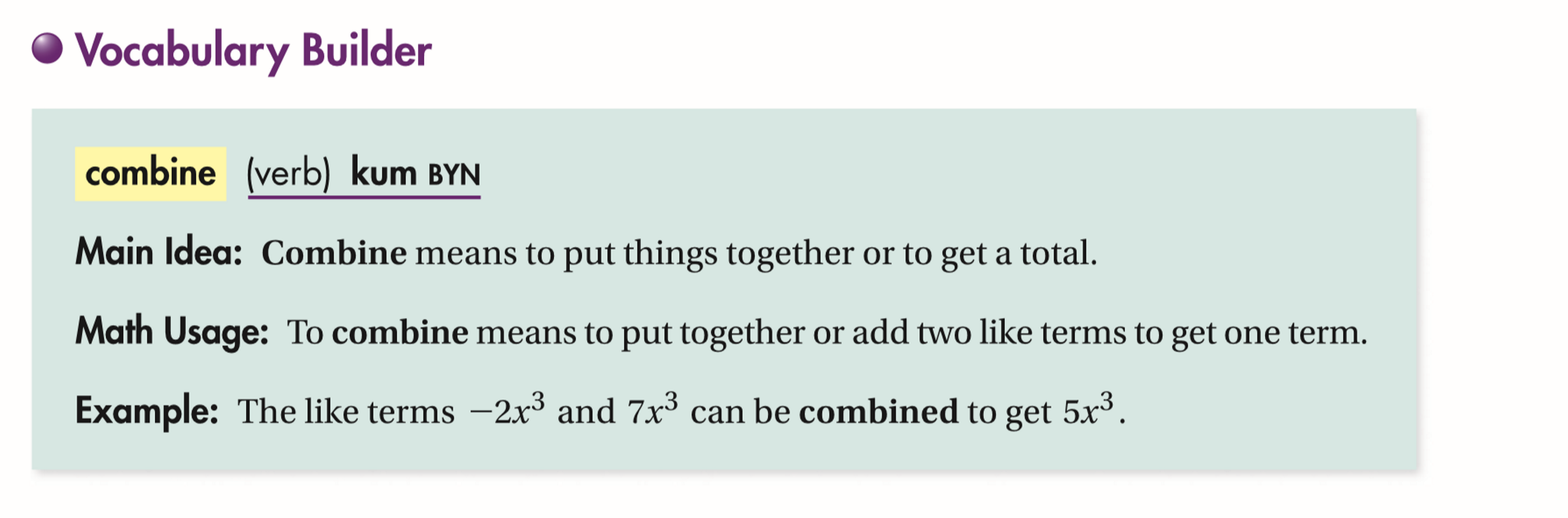25
10 pts
Use Your Vocabulary: Which expression shows the expression below with like terms combined ?26
100 pts
Notes: Take a clear picture or screenshot of your Cornell notes for this lesson. Upload it to the canvas. Zoom and pan as needed.

For a refresher on the Cornell note-taking system, click here.
27
10 pts
Reflection: Math Success
Add to my formatives list

Formative uses cookies to allow us to better understand how the site is used. By continuing to use this site, you consent to the Terms of Service and Privacy Policy.